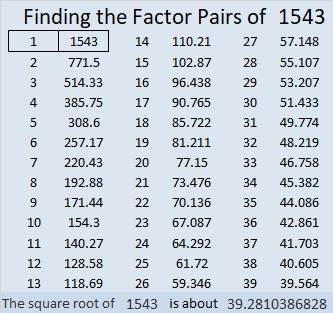# 1543 Another Letter

### Today’s Puzzle:

My goal was to make this next letter of my message using as few clues in the puzzle as possible. You now have enough clues to solve this puzzle and know my message to you.### Factors of 1543:

• 1543 is a prime number.
• Prime factorization: 1543 is prime.
• 1543 has no exponents greater than 1 in its prime factorization, so √1543 cannot be simplified.
• The exponent in the prime factorization is 1. Adding one to that exponent we get (1 + 1) = 2. Therefore 1543 has exactly 2 factors.
• The factors of 1543 are outlined with their factor pair partners in the graphic below.How do we know that 1543 is a prime number? If 1543 were not a prime number, then it would be divisible by at least one prime number less than or equal to √1543. Since 1543 cannot be divided evenly by 2, 3, 5, 7, 11, 13, 17, 19, 23, 29, 31, or 37, we know that 1543 is a prime number.

### One More Fact about the Number 1543:

1543 is the sum of consecutive numbers as well as the difference of those same consecutive numbers but squared:
771 + 772 = 1543;
772² – 771² = 1543.
(1543 has that property because it’s an odd number greater than 1.)